# Class 9 NCERT Solutions- Chapter 11 Constructions – Exercise 11.2

• Last Updated : 28 Dec, 2020

### Question 1. Construct a ∆ ABC in which BC = 7 cm, ∠B = 75° and AB + AC = 13 cm.

Solution:

Steps of construction:

Attention reader! All those who say programming isn't for kids, just haven't met the right mentors yet. Join the  Demo Class for First Step to Coding Coursespecifically designed for students of class 8 to 12.

The students will get to learn more about the world of programming in these free classes which will definitely help them in making a wise career choice in the future.

1. Draw a line segment BC base of cm is drawn.
2. At point B draw an angle of 75°.
3. Cut BD =13cm from BY.
4. Join ∠D which intersect BD at A.
5. Join AC. Now triangle ABC is the required triangle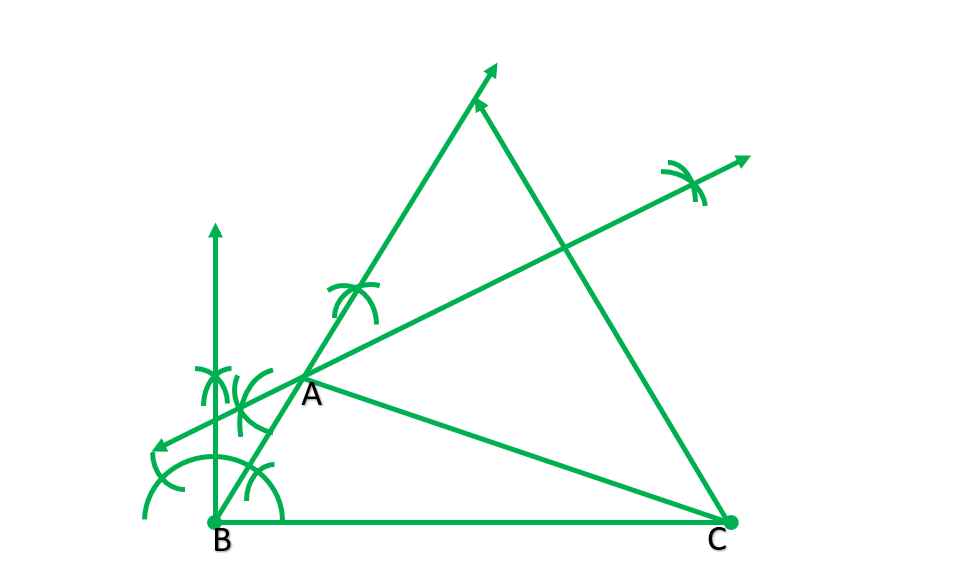### Question 2. Construct a ABC in which BC = 8 cm, ∠B = 45° and AB – AC = 35 cm.

Solution:

Steps of construction:

1. Draw  a line segment BC=8cm.
2. At point B, draw angle 45°.
3. Cut BD=3.5 from BY.
4. Join CD.
5. Draw perpendicular bisector of CD, which construct BY at A.
6. Join AC. NOW, ABC is the required triangle.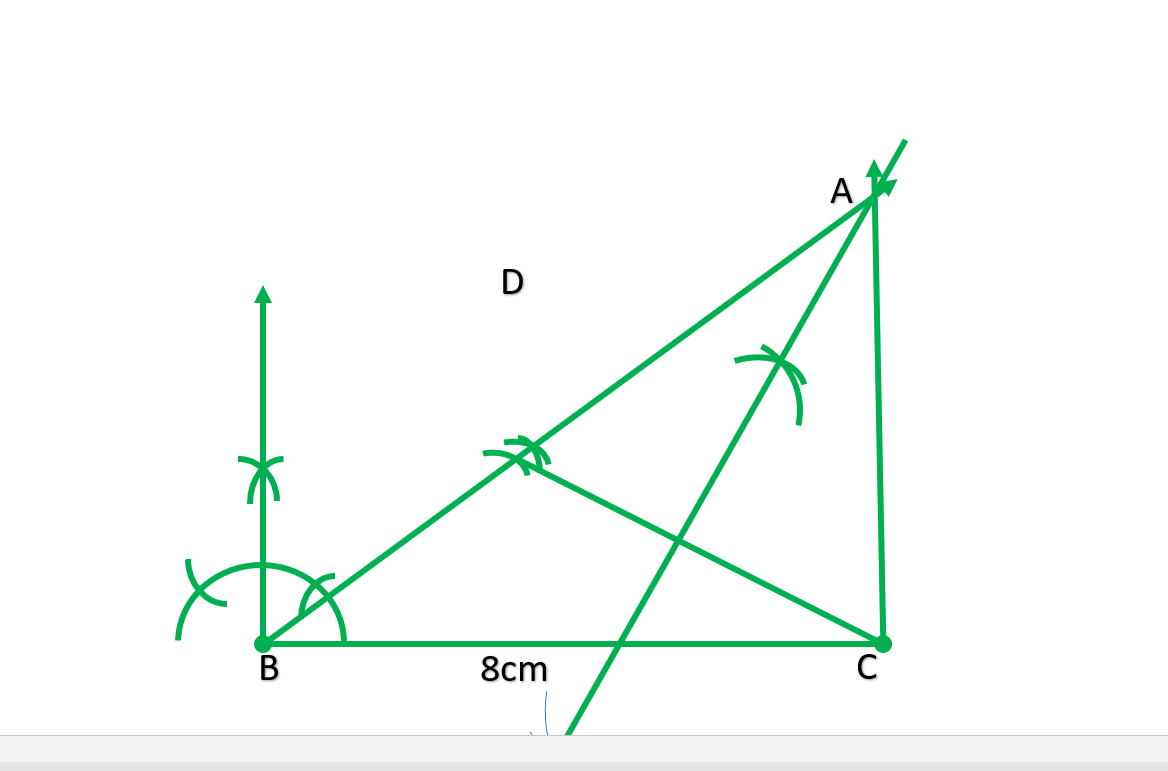### Question 3. Construct a ∆ ABC in which QR = 6 cm, ∠Q = 60° and PR – PQ = 2 cm.

Solution:

Steps of construction:

1. Draw a line segment QR=6cm.
2. At point Q draw angle 60°.
3. Extend PQ to Y’.
4. Cut QS =2cm from QY’.
5. Join RS.
6. Draw perpendicular bisector of RS which intersect QY at P.
7. Join PR. Now, PQR is the required triangle.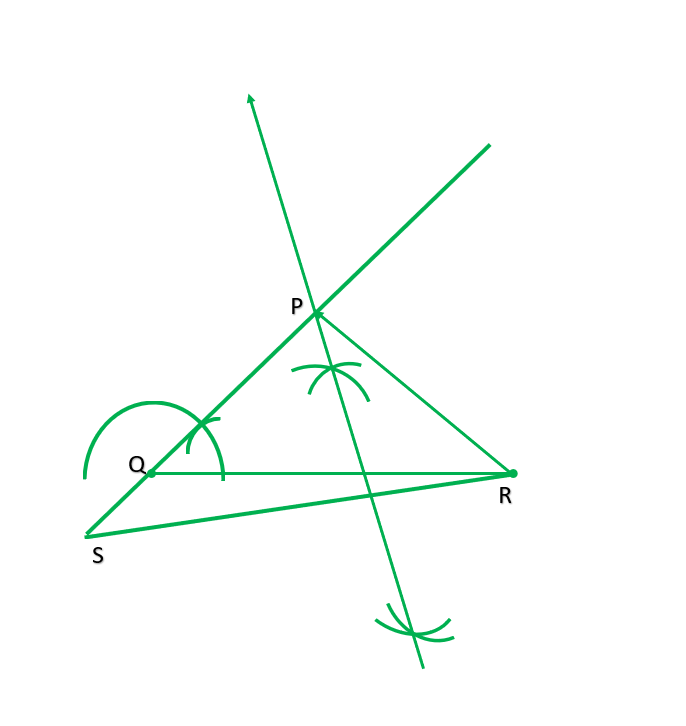### Question 4. Construct a ∆ XYZ in which ∠Y = 30°, ∠Y = 90° and XY + YZ + ZX = 11 cm.

Solution:

Steps of construction:

1. Draw a line segment AB=11cm.
2. At point A draw ∠BAP=30°.
3. At point B draw angle 90°.
4. Draw the bisector of ∠BAP and ∠ABR which intersect each other at X.
5. Join AX and BX.
6. Draw perpendicular bisector of AX and BX which intersect AB on Y and Z respectively.
7. Join XY and XZ. Then XYZ is the required triangle.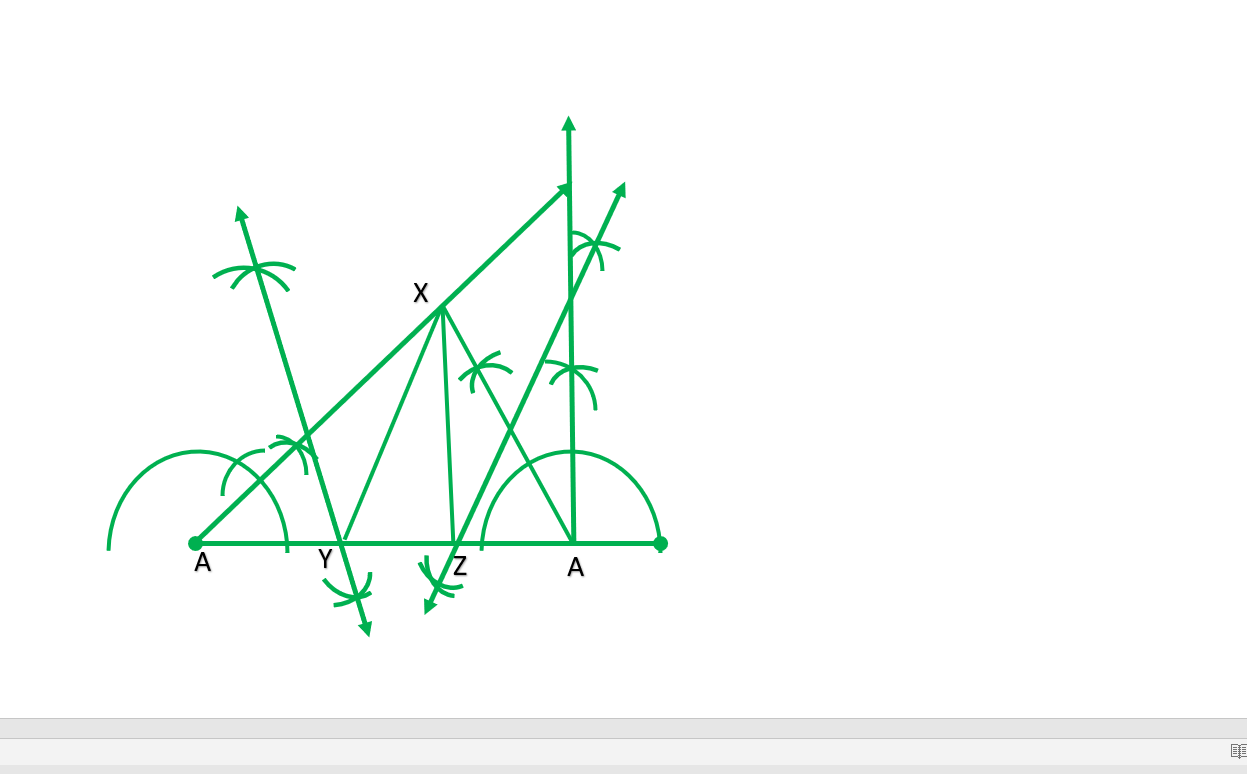### Question 5. Construct a right triangle whose base is 12 cm and sum of its hypotenuse and other side is 18 cm.

Solution:

Steps of construction:

1. Draw a line segment of BC=12cm.
2. At point B draw angle b=90°
3. Cut BD =18cm.
4. Join CD.
5. Draw perpendicular bisector of CD which intersect BD at point A.
6. Join AC. Now ABC is the required triangle.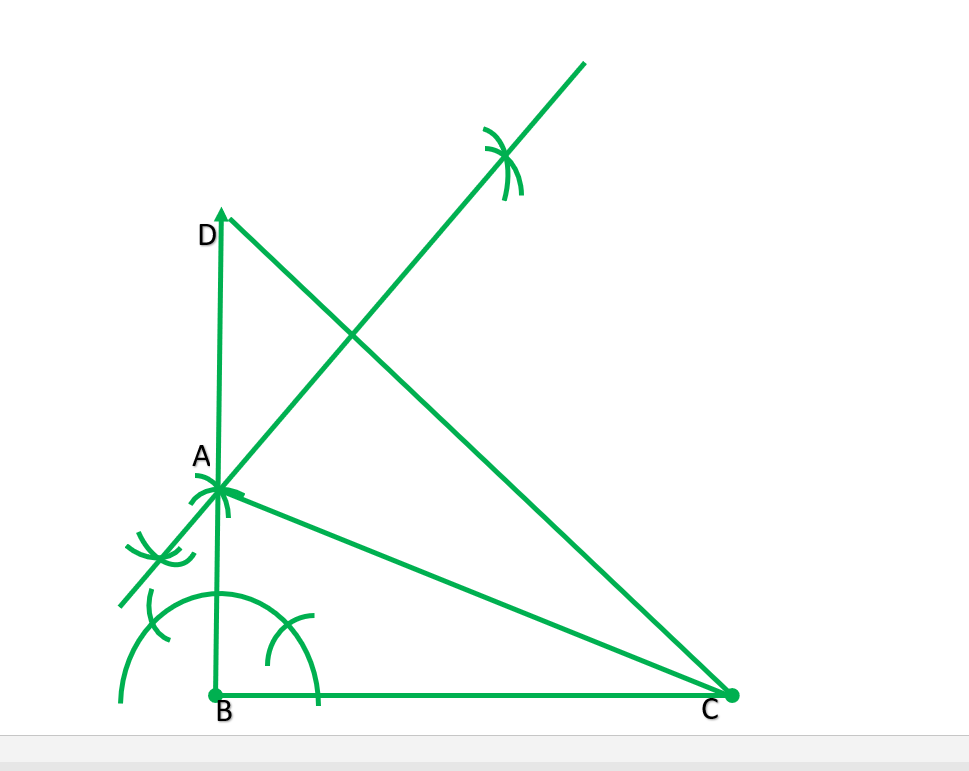My Personal Notes arrow_drop_up